Question

If the energy of the system is constant, and all forces are conservative, which statement is...

If the energy of the system is constant, and all forces are conservative, which statement is true:

 a. KEi + PEi = KEf +PEf b. KEi + KEf = PEi + PEf c. ΔKE = ΔPE d. PEi = PEf

If only conservative forces are involved in the system and internal energy of system is constant than sum of total initial energy is equal to sum of total final energy.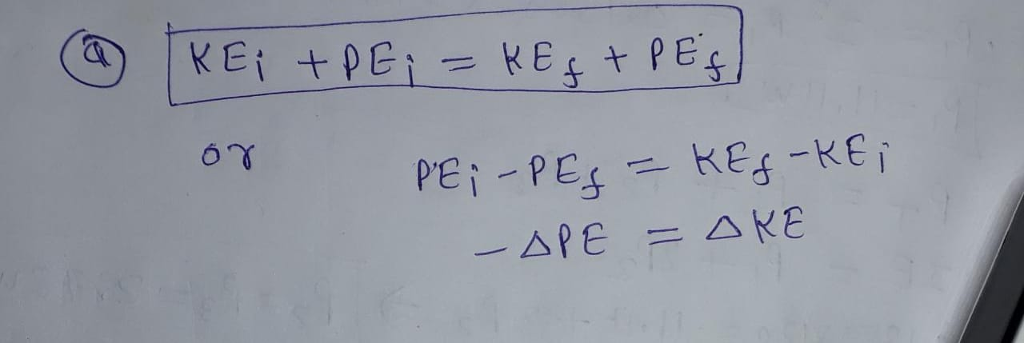Earn Coins

Coins can be redeemed for fabulous gifts.

Similar Homework Help Questions
• 3.1 Determine which of the following forces are conservative by find- ing the curl: (a) F...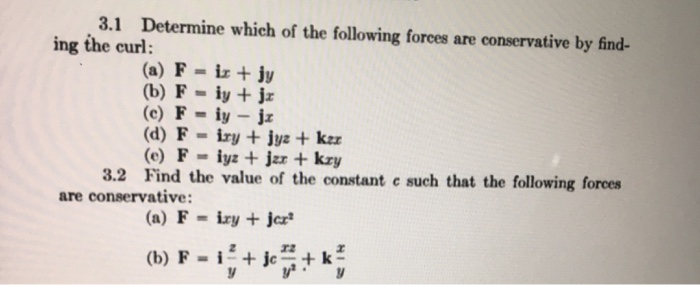3.1 Determine which of the following forces are conservative by find- ing the curl: (a) F ix + jy (b) F - iy + jx (C) F - iy - jz (d) F - iry + jyz + kzz (e) F-iyz + jar + kry 3.2 Find the value of the constant c such that the following forces are conservative: (a) F = ixy + jer (b) F = i + jcm+

• under what conditions is mechanical energy conserved? only when conservative forces are present Only when the...

under what conditions is mechanical energy conserved? only when conservative forces are present Only when the net talk on a system is zero only when the net force on the system is zero mechanical Everly is always conserved

• QUESTIONS At constant pressure and temperature, which statement is true? A. All reactions for which AH<0...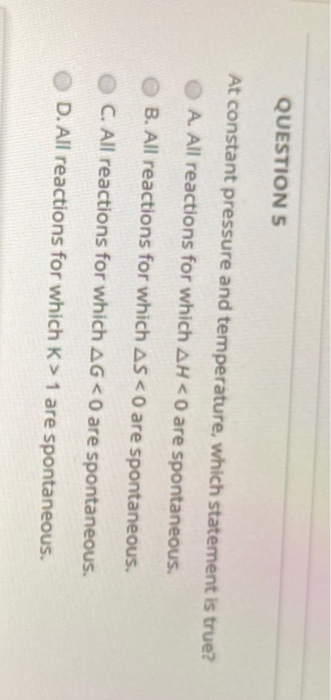QUESTIONS At constant pressure and temperature, which statement is true? A. All reactions for which AH<0 are spontaneous B. All reactions for which ASKO are spontaneous. C. All reactions for which AG<0 are spontaneous. D. All reactions for which K> 1 are spontaneous.

• HELP!! mastering physics: 7.2: Conservation of Energy with Conservative Forces

Learning Goal: To practice Problem-SolvingStrategy 7.2 Conservation of energy with conservative forces.A basket of negligible weight hangs from a vertical spring scale offorce constant1500 . If you suddenly put an adobebrick of mass3.00 in the basket, find the maximum distance thatthespring will stretch.Problem-Solving Strategy 7.2 Conservation of energy with conservativeforcesSET UPIdentify the system youwill analyze, and decide on the initial and final states (positionsand velocities) you will use in solving the problem. Draw oneormore sketches showing the initial and finalstates.Define...

• Conservative Forces and Potential Energy? A simple Atwood's machine uses two masses, m1 and m2. Starting...

Conservative Forces and Potential Energy? A simple Atwood's machine uses two masses, m1 and m2. Starting from rest, the speed of the two masses is 6 m/s at the end of 5 s. At that instant, the kinetic energy of the system is 67 J and each mass has moved a distance of 15 m. Determine the values of m1 and m2

• 3. A particle subject only to conservative forces has the potential energy vs. position curve shown...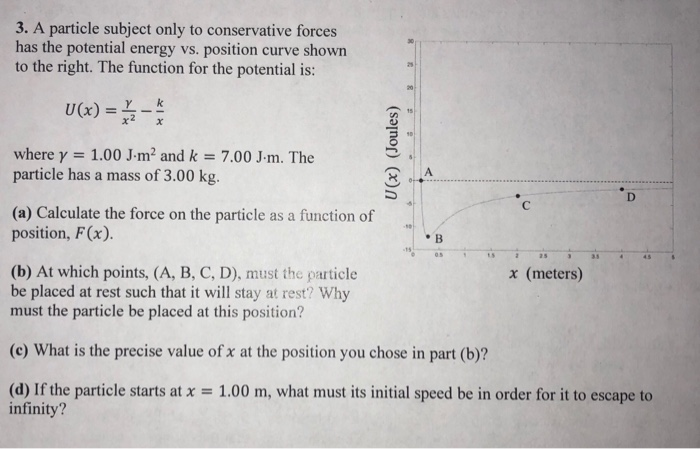3. A particle subject only to conservative forces has the potential energy vs. position curve shown to the right. The function for the potential is: U(x)-k where γ 1.00 J.m2 and k-7.00 Jr. The particle has a mass of 3.00 kg. (a) Calculate the force on the particle as a function of position, F(x). (b) At which points, (A, B, C, D), must the particle be placed at rest such that it will stay at rest? Why must the particle...

• An object is moving forward with a constant velocity. Which statement about this object MUST be...

An object is moving forward with a constant velocity. Which statement about this object MUST be true? A. No forces are acting on the object. B. The net force on the object is in the forward direction. C. The acceleration of the object is in the forward direction. D. The net force on the object is zero.

• 30) At constant pressure and temperature, which statement is true? A) All reactions for which k...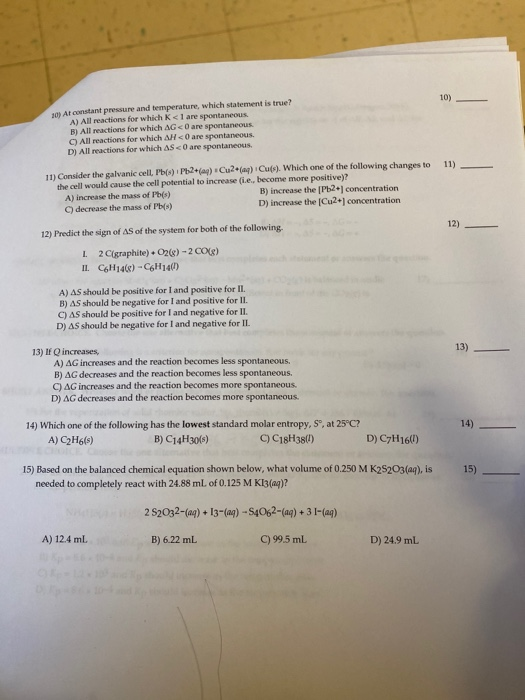30) At constant pressure and temperature, which statement is true? A) All reactions for which k < 1 are spontaneous B) All reactions for which AG are spontaneous C) All reactions for which AH<0 are spontaneous. D) All reactions for which AS <0 are spontaneous 11) 11) Consider the galvanic cell, Pb(s) IP20) Cu2+(a) Cu(s). Which one of the following changes to the cell would cause the cell potential to increase (ie, become more positive)? A) increase the mass of...

• Question 11 Ip Which statement is true of the internal energy (AU) of a system and...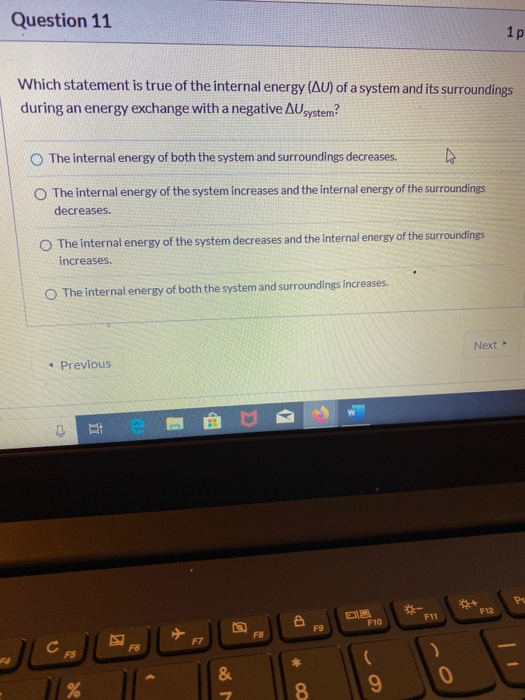Question 11 Ip Which statement is true of the internal energy (AU) of a system and its surroundings during an energy exchange with a negative Ausystem? The internal energy of both the system and surroundings decreases The internal energy of the system increases and the internal energy of the surroundings decreases. The internal energy of the system decreases and the internal energy of the surroundings increases. The internal energy of both the system and surroundings increases. Next → Previous CES...

• 9.09 kg particle is subjected to only conservative forces and its potential energy diagram is shown...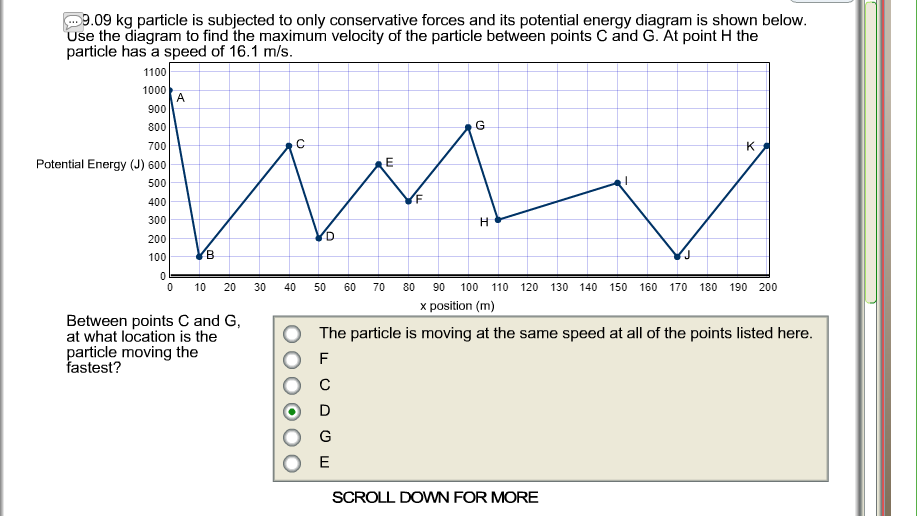9.09 kg particle is subjected to only conservative forces and its potential energy diagram is shown below se the diagram to find the maximum velocity of the particle between points C and G. At point H the particle has a speed of 16.1 m/s 1000 A 900 800 700 Potential Energy (J) 600 500 400 300 200 100 0 10 20 30 40 50 60 70 80 90 100 110 120 130 140 150 160 170 180 190 200 x...